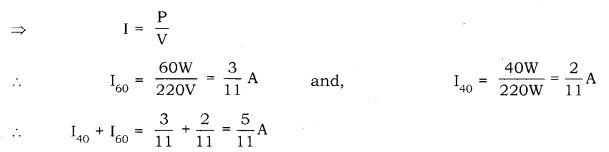# Two lamps, one related 60w at 220w at 220V and the other 40w at 220V

Two lamps, one related 60w at 220w at 220V and the other 40w at 220V, are connected in parallel to the electric supply at 220V.

1. Draw a circuit diagram to show the connections.
2. Calculate the current drawn from the electric supply.
3. Calculate the total energy consumed by the two lamps together when they operate for one hour.

1.2.Electric current flowing through a bulb =Therefore, total current flowing through the circuit = A = 0.45 A

3.Total energy consumed = Power × Time
That is, E = P × f = (40W + 60W) × 1h = 100Wh = 0.1 kWh.